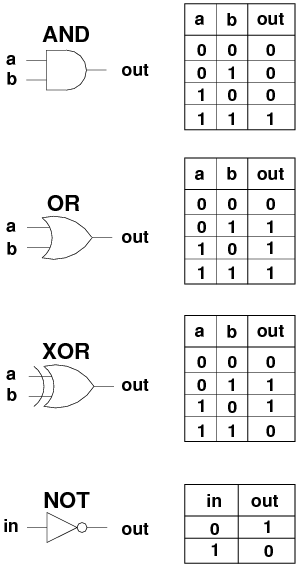# Binary Numbers from a Decimal Perspective

Decimal numbers have a base of 10, meaning that any decimal number can be represented as the sum of powers of 10.

# Boolean Algebra

A bitwise operators &(AND), |(OR), ^(XOR), and ~(NOT) are operations used in boolean algebra. They are logical operators that compare variables that can only have two discrete values: true or false. I like to visualize them as functions:These are logic gates. Don’t worry about their structure, just note the number of inputs and outputs.

# Bitwise Operations

A Bitwise operation is just an operation that is performed bit by bit.

# Bitwise Shift Operators

The operators ‘<<’ ‘>>’ and ‘>>>’ all work similar to an array shift/unshift. They push digits up or down the number. You must specify a number of digits to shift.

# Why Use Bitwise Operators

A simple answer would be that you may not, in practice. Bitwise operations are incredibly efficient, but they make code harder to understand. For the same reason that we strive to create higher level programming languages, we tend to sacrifice small performance benefits for readability.